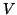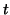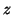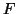Next: Examples Up: tr01cb1 Previous: Estimation of Variance Components

# Limitations

In this section we discuss the limitations of the model framework presented above. The first and most obvious limitation is that the framework is purely linear, as it is an instance of the GLM. Linear modelling, however, is a very common and flexible model for FMRI data. Secondly, in order for the model to be decomposed into two levels, and hence allow for efficient two-level computation, it is necessary that the stacked first-level covariance matrix,, does not contain any non-zero off-diagonal blocks. This is equivalent to assuming that the first-level residuals are not correlated between sessions or subjects.

Efficient computation at the second-level requires full access to the first-level parameter estimates and associated covariances. This involves both the variances of the parameter estimates and the covariances between different parameters. It is not sufficient to only use the first-level statistical parametric maps (i.e.-scores,-scores or-scores). Finally, the estimation of covariance parameters at the second-level often imposes restrictions in the types of model which are practically estimable. This is often more problematic at the second level because there is usually only a small number of subjects/sessions that are being modelled. For instance, while it is possible to formulate a model where the variance about the group mean is different for each session/subject, such a model is not estimable because there is only a single measurement per session/subject.Next: Examples Up: tr01cb1 Previous: Estimation of Variance Components
Christian Beckmann 2003-07-16